# 1 Indices to assess riverscape connectivity

The riverconn package is used to calculate indices for river network connectivity. For a review of the indices, see Jumani et al., 2021, while for a list of the functionalities of the package and its architecture, see Baldan et al., 2022.

If you use this package, cite it as: https://doi.org/10.1016/j.envsoft.2022.105470.

For a tutorial on how to generate graphs representing rivers from “real world” data see: https://damianobaldan.github.io/riverconn_tutorial/

## 1.1 The river network as a graph

This package implements algorithms to compute commonly used indices to assess river networks connectivity All those indices assume a conceptualization of the river networks as a graph $$L = (E,V)$$, where vertices (nodes) $$V$$ represent single reaches, and edges (links) $$E$$ represent either confluences or longitudinal barriers.

For example, the graphs below represents a river with ten reaches. The graph on the left is directed, i.e. edges are defined for ordered pair of vertices. The graph on the right is undirected, as the order of vertices for the definition of edges is unimportant. Both graph have a ‘tree-like’ structure, since no loops exist (acyclic graphs): this structure can be used to describe a river system. In both examples, both barriers and confluences are present. The edges between nodes 1 and 2 and 3 and 2 are confluences. The edge between node 2 and 4 is a barrier.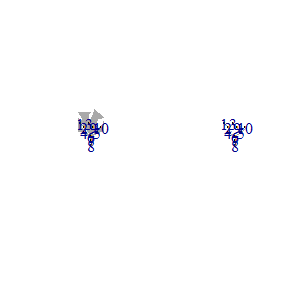## 1.2 Generalized riverscape connectivity index

river networks-level connectivity can be expressed in terms of coincidence probability (Pascual-Hortal and Saura, 2006), i.e. the probability that two random points in a river network are connected. Once the dispersal probability $$I_{ij}$$ is defined for each couple of $$i,j$$ nodes in the graph, generalized connectivity indices for catchment and reach scales can be calculated.

### 1.2.1 Catchment Connectvity index

The catchment-scale connectivity index (CCI) is calculated as:

$\ CCI = \sum_{i = 1}^{n} \sum_{j = 1}^{n} I_{ij} \frac{w_i w_j}{W^2}$ Where $$w_i$$ and $$w_j$$ are some node-level attributes (weights), and $$W$$ is the sum of sum of the nodes weights for the whole river networks.

### 1.2.2 Reach Connectivity Index

The reach-scale connectivity index (RCI) is calculated by limiting the summation to all the connections to the single node $$i$$. $\ RCI_i = \sum_{j = 1}^{n} I_{ij} \frac{w_j}{W}$ Where $$w_j$$ are some node-level attributes (weights), and $$W$$ is the sum of sum of the nodes weights for the whole river networks.

### 1.2.3 Node weights

Nodes weights can be arbitrarily chosen. Common features used are the reach length $$l_i$$, area $$A_i$$, or volume $$V_i$$. Alternatively, the habitat suitability index (HSI) can be used, defined as the ratio of length/area that is suitable for a specific organism. $\ HSI_i = \frac{{l}_{i,suitable}}{l_i}$ Here $${l}_{i,suitable}$$ is the fraction of reach length of reach $$i$$ that are suitable, and are usually referred to as weighted suitable length or weighted suitable area.

## 1.3 Dispersal probability

The dispersal probability depends on several factors: the presence of barriers between nodes $$i$$ and $$j$$ , the presence of suitable habitats in nodes $$i$$ and $$j$$ and alongside the connection, and the distance between $$i$$ and $$j$$. The dispersal probability $$I_{ij}$$ is thus determined by several contributions. Those contributions are multiplied:

$\ I_{ij} = c_{ij}B_{ij}$ where $$c_{ij}$$ accounts for the structural connectivity, i.e. it depends exclusively on the presence of barriers between nodes $$i$$ and $$j$$, and $$B_{ij}$$ accounts for the functional connectivity, i.e. it depends exclusively on the distance and the organisms movement/dispersal abilities.

### 1.3.1 Structural connectivity

The structural connectivity depends on the presence of barriers between nodes $$i$$ and $$j$$, and can be expressed as a function of the types of barriers present in the path expressed as a sequence of passability values. The passability $$p_m$$ for the $$m$$-th barrier is defined as the probability that the reaches immediately upstream and downstream the barrier $$m$$ are connected.

If the flow directionality is not relevant (i.e. the river graph can be conceptualized as undirected),

$\ c_{ij} = \prod_{m = 1}^{k} p_m^u p_m^d$ Where the product extends over the $$k$$ nodes that are part of the path connecting reaches $$i$$ and $$j$$, $$p_m^u$$ is the upsstream passability of the $$m$$-th barrier and $$p_m^u$$ is the upstream passability of the $$m$$-th barrier. This definition based solely on products yields a symmetric coincidence probability (i.e. $$c_{ij} = c_{ji}$$).

A directional version of $$c_{ij}$$ can be defined as: $\ c_{ij} = \prod_{m = 1}^{k} p_m^{eq}$ $p_m^{eq} = \begin{cases} p_m^u & \mbox{if barrier m is encountered moving upstream in the path from i to j } \\ p_m^d & \mbox{if barrier m is encountered moving downstream in the path from i to j } \end{cases}$ If $$i$$ and $$j$$ are located in different sub-catchments, the path from i to j will be moving downstream in some sections and upstream in some other secions: this $$p_m^{eq}$$ definition ensures the retained passability value is consistent with the directionality of the path from $$i$$ to $$j$$ (i.e. $$c_{ij} \ne c_{ji}$$)

### 1.3.2 Functional connectivity

Functional connectivity can be calculated as a function of the distance between reaches. An exponential dispersal kernel can be used:

$\ B_{ij} = PD^{d_{ij}}$ where $$PD$$ is in the $$(0,1)$$ interval (smaller values mean more restricted movement), and $$d_{ij}$$ is the distance between reaches $$i$$ and $$j$$. Alternatively, a threshold based probability can be used: $\ B_{ij} = \begin{cases} 0 & \mbox{when } d_{ij}>d_{tr} \\ 1 & \mbox{when } d_{ij}<=d_{tr} \end{cases}$ Both definitions can be easily adapted to asymmetric dispersal by defining $$PD_{d}$$, $$PD_{u}$$, $$d_{tr,u}$$, and $$d_{tr,d}$$, and calculating $$B_{ij} = B_{ij}^u B_{ij}^d$$ where $$B_{ij}^u$$ and $$B_{ij}^d$$ are the index $$B_{ij}$$ contribution calculated for the ‘downstream moving’ and ‘upstream moving’ sections in the path from reach $$i$$ to $$j$$.

The distance $$d_{ij}$$ can be either the geometric distance, or any other measure of effective distance (e.g. $$d_{ij} / (1-{HSI}_{ij})$$ provides an estimate of effective distance that depends on the habitat suitability index between reaches $$i$$ and $$j$$)

## 1.4 Prioritization of barriers

All the defined connectivity index can be used to prioritize barriers removal with a ‘leave-one-out’ approach. For each barrier, the index $$dCCI$$ can be defined as: $\ dCCI_{m} = 100 \frac{CCI - CCI_{m, removed}}{GCI}$ where $$CCI$$ is the generalized connectivity index calculated for the original river networks with all the barriers implemented, and CCI_{m, removed} is the index recalculated when barrier $$m$$ is removed or its passability is changed (an equivalent for the reach scale, $$dRCI_{i}$$, can be defined similarly) .

An alternative version of the index for prioritizing barriers can be calculated as the decrease in river networks connectivity after a single barrier is implemented, with a ‘add-one’ approach.

## 1.5 Time-dependent connectivity

When barriers metadata on the year of construction and the year of implementation of mitigation measures are available, a time trajectory of GCI can be computed (e.g. Segurado et al., 2013).

# 2 Preprocessing of input data

This package relies heavily on the functionalities of the igraph package. The igraph package implements routines for simple graphs and network analysis. It can handle large graphs very well and provides functions for generating random and regular graphs, graph visualization, centrality methods and much more. The package allows for easy construction of igraph objects based on edges and vertices lists or adjacency matrices. The book ‘Statistical Analysis of Network Data with R’ by Kolaczyk and Csardi (2014) offers a comprehensive tutorial on the possibilities offered by the ‘igraph’ package.

A more comprehensive tutorial, including a real-world case study can be found here: https://damianobaldan.github.io/riverconn_tutorial/

## 2.1 Preliminary steps

library(igraph)
library(dplyr)
library(tidyr)
library(ggnetwork)
library(viridis)
library(riverconn)
library(doParallel)

## 2.2 Input class ‘igraph’ object

All the functions implemented in this package use as main input an object of class igraph. There are different ways an object of class igraph can be created. A symbolic sequence of edges can be used with the function graph_from_literal for small, toy graphs.

g <- graph_from_literal(1-+2, 2-+5, 3-+4, 4-+5, 6-+7, 7-+10, 8-+9, 9-+10,
5-+11, 11-+12, 10-+13, 13-+12, 12-+14, 14-+15, 15-+16)
g
## IGRAPH 86e8a11 DN-- 16 15 --
## + attr: name (v/c)
## + edges from 86e8a11 (vertex names):
##   1 ->2  2 ->5  5 ->11 3 ->4  4 ->5  6 ->7  7 ->10 10->13 8 ->9  9 ->10
##  11->12 12->14 13->12 14->15 15->16

Note that when a graph is defined this way, edged and vertices attributes are not defined.

# Edges
E(g)
## + 15/15 edges from 86e8a11 (vertex names):
##   1 ->2  2 ->5  5 ->11 3 ->4  4 ->5  6 ->7  7 ->10 10->13 8 ->9  9 ->10
##  11->12 12->14 13->12 14->15 15->16

# vertices
V(g)
## + 16/16 vertices, named, from 86e8a11:
##   1  2  5  3  4  6  7  10 8  9  11 12 13 14 15 16

The graph can be converted to data frame with the function as_data_frame, specifying if edges or vertices are to be exported. Accordingly, the function graph_from_data_frame can be used to create an igraph object from a data frame.

igraph::as_data_frame(g, what = "edges")
##    from to
## 1     1  2
## 2     2  5
## 3     5 11
## 4     3  4
## 5     4  5
## 6     6  7
## 7     7 10
## 8    10 13
## 9     8  9
## 10    9 10
## 11   11 12
## 12   12 14
## 13   13 12
## 14   14 15
## 15   15 16

igraph::as_data_frame(g, what = "vertices")
##    name
## 1     1
## 2     2
## 5     5
## 3     3
## 4     4
## 6     6
## 7     7
## 10   10
## 8     8
## 9     9
## 11   11
## 12   12
## 13   13
## 14   14
## 15   15
## 16   16

Finally, an igraph object can be exported to and generated from adjacency matrices using the functions as_adjacency_matrix and graph_from_adjacency_matrix, specifying if edges or vertices are to be exported.

igraph::as_adjacency_matrix(g)
## 16 x 16 sparse Matrix of class "dgCMatrix"
##
## 1  . 1 . . . . . . . . . . . . . .
## 2  . . 1 . . . . . . . . . . . . .
## 5  . . . . . . . . . . 1 . . . . .
## 3  . . . . 1 . . . . . . . . . . .
## 4  . . 1 . . . . . . . . . . . . .
## 6  . . . . . . 1 . . . . . . . . .
## 7  . . . . . . . 1 . . . . . . . .
## 10 . . . . . . . . . . . . 1 . . .
## 8  . . . . . . . . . 1 . . . . . .
## 9  . . . . . . . 1 . . . . . . . .
## 11 . . . . . . . . . . . 1 . . . .
## 12 . . . . . . . . . . . . . 1 . .
## 13 . . . . . . . . . . . 1 . . . .
## 14 . . . . . . . . . . . . . . 1 .
## 15 . . . . . . . . . . . . . . . 1
## 16 . . . . . . . . . . . . . . . .

## 2.3 Decorating the class ‘igraph’ object

Once the structure of the network is defined, the graph can be decorated with edges and vertices attributes. Attributes can be either added directly to the graph or joined to the edges and vertices data frame. edges and vertices attributes are saved as vectors, so common, data.frame-like operations are possible.

Here we add the dam information as edge attribute, including the field ‘id_dam’, and the reach information data as vertices attributes, including the length and the corresponding habitat suitability index.

# Decorate edges
E(g)$id_dam <- c("1", NA, "2", "3", NA, "4", NA, "5", "6", NA, NA, NA, NA, "7", NA) E(g)$type <- ifelse(is.na(E(g)$id_dam), "joint", "dam") E(g) ## + 15/15 edges from 86e8a11 (vertex names): ##  1 ->2 2 ->5 5 ->11 3 ->4 4 ->5 6 ->7 7 ->10 10->13 8 ->9 9 ->10 ##  11->12 12->14 13->12 14->15 15->16 # Decorate vertices V(g)$length <- c(1, 1, 2, 3, 4, 1, 5, 1, 7, 7, 3, 2, 4, 5, 6, 9)
V(g)$HSI <- c(0.2, 0.1, 0.3, 0.4, 0.5, 0.5, 0.5, 0.6, 0.7, 0.8, 0.8, 0.8, 0.8, 0.8, 0.8, 0.8) V(g)$Id <- V(g)\$name
V(g)
## + 16/16 vertices, named, from 86e8a11:
##   1  2  5  3  4  6  7  10 8  9  11 12 13 14 15 16

## 2.4 Visualizing the ‘igraph’ object

The ggnetwork library can be used to plot a igraph object with tidyverse-friendly functions. The function ggnetwork is used to fortify igraph objects with a specified layout. Here the tree layout is used, but for real river networks the coordinates of the reaches centroids can be used.

gg0 <- ggnetwork(g, layout =  layout_as_tree(g %>% as.undirected, root = 16), scale = FALSE)

ggplot(gg0, aes(x = x, y = y, xend = xend, yend = yend)) +
geom_nodes(alpha = 0.3) +
geom_edges(alpha = 0.5,
arrow = arrow(length = unit(10, "pt"), type = "closed"),
aes(color = type)) +
scale_color_viridis(discrete = TRUE)+
geom_nodetext(aes(label = name), fontface = "bold") +
theme_blank()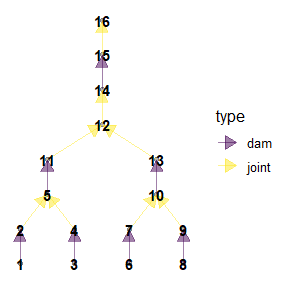This function can be used to display all the graph attributes.

ggplot(gg0, aes(x = x, y = y, xend = xend, yend = yend)) +
geom_edges(alpha = 0.5,
arrow = arrow(length = unit(10, "pt"), type = "open")) +
geom_nodes(aes(size = length, color = HSI)) +
scale_color_viridis()+
theme_blank()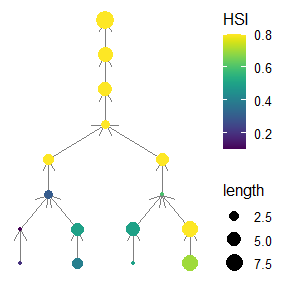## 2.5 Assigning network directionality

The riverconn package implements the function set_graph_directionality that allows to assign the directionality of the graph once an outlet is defined.

oldpar <- par(mfrow = c(1,1))
par(mfrow=c(1,3))
g1 <- set_graph_directionality(g, field_name = "Id", outlet_name = "16")
g2 <- set_graph_directionality(g, field_name = "Id", outlet_name = "5")
plot(as.undirected(g), layout = layout_as_tree(as.undirected(g), root = 8, flip.y = FALSE))
plot(g1, layout = layout_as_tree(as.undirected(g1), root = 16, flip.y = FALSE))
plot(g2, layout = layout_as_tree(as.undirected(g2), root = 5, flip.y = FALSE))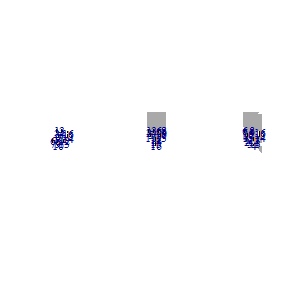par(oldpar)

# 3 Indices calculation

The function index_calcualtion is used to calculate all the nuances of the CCI and RCI

Before calculation, the information on the barriers passability are needed.

# Check edged and nodes attributes, add pass_u and pass_d fields
g_v_df <- igraph::as_data_frame(g, what = "vertices")
g_v_df
##    name length HSI Id
## 1     1      1 0.2  1
## 2     2      1 0.1  2
## 5     5      2 0.3  5
## 3     3      3 0.4  3
## 4     4      4 0.5  4
## 6     6      1 0.5  6
## 7     7      5 0.5  7
## 10   10      1 0.6 10
## 8     8      7 0.7  8
## 9     9      7 0.8  9
## 11   11      3 0.8 11
## 12   12      2 0.8 12
## 13   13      4 0.8 13
## 14   14      5 0.8 14
## 15   15      6 0.8 15
## 16   16      9 0.8 16
g_e_df <- igraph::as_data_frame(g, what = "edges") %>%
mutate(pass_u = ifelse(!is.na(id_dam),0.1,NA),
pass_d = ifelse(!is.na(id_dam),0.7,NA))
g_e_df
##    from to id_dam  type pass_u pass_d
## 1     1  2      1   dam    0.1    0.7
## 2     2  5   <NA> joint     NA     NA
## 3     5 11      2   dam    0.1    0.7
## 4     3  4      3   dam    0.1    0.7
## 5     4  5   <NA> joint     NA     NA
## 6     6  7      4   dam    0.1    0.7
## 7     7 10   <NA> joint     NA     NA
## 8    10 13      5   dam    0.1    0.7
## 9     8  9      6   dam    0.1    0.7
## 10    9 10   <NA> joint     NA     NA
## 11   11 12   <NA> joint     NA     NA
## 12   12 14   <NA> joint     NA     NA
## 13   13 12   <NA> joint     NA     NA
## 14   14 15      7   dam    0.1    0.7
## 15   15 16   <NA> joint     NA     NA

# Recreate graph
g <- igraph::graph_from_data_frame(d = g_e_df, vertices = g_v_df)
g
## IGRAPH 887c0f3 DN-- 16 15 --
## + attr: name (v/c), length (v/n), HSI (v/n), Id (v/c), id_dam (e/c),
## | type (e/c), pass_u (e/n), pass_d (e/n)
## + edges from 887c0f3 (vertex names):
##   1 ->2  2 ->5  5 ->11 3 ->4  4 ->5  6 ->7  7 ->10 10->13 8 ->9  9 ->10
##  11->12 12->14 13->12 14->15 15->16

Index with default settings.

index_calculation(g, param = 0.9)
##        num  den    index
## 1 553.8299 3721 0.148839

Index with default settings, only $$c_{ij}$$ or $$B_{ij}$$ contributions

index_calculation(g, B_ij_flag = FALSE)
##        num  den     index
## 1 791.8553 3721 0.2128071
index_calculation(g, param = 0.9, c_ij_flag = FALSE)
##       num  den     index
## 1 1200.63 3721 0.3226632

Index with default settings, only $$B_{ij}$$ contributions with threshold on the distance

index_calculation(g, c_ij_flag = FALSE,
dir_distance_type = "asymmetric",
disp_type = "threshold", param_u = 0, param_d = 5)
##   num  den     index
## 1 453 3721 0.1217415
index_calculation(g, c_ij_flag = FALSE,
dir_distance_type = "asymmetric",
disp_type = "threshold", param_u = 5, param_d = 10)
##    num  den     index
## 1 1028 3721 0.2762698
index_calculation(g, c_ij_flag = FALSE,
dir_distance_type = "symmetric",
disp_type = "threshold", param = 10)
##    num  den    index
## 1 1239 3721 0.332975

Index for reach, inbound connections used, only $$B_{ij}$$ contributions with threshold on the distance

index_calculation(g, c_ij_flag = FALSE,
index_type = "reach", index_mode = "to",
dir_distance_type = "asymmetric",
disp_type = "threshold", param_u = 0, param_d = 5)
##    name num den      index
## 1     1   7  61 0.11475410
## 2     2   6  61 0.09836066
## 5     5   7  61 0.11475410
## 3     3   7  61 0.11475410
## 4     4   6  61 0.09836066
## 6     6   6  61 0.09836066
## 7     7   6  61 0.09836066
## 10   10   7  61 0.11475410
## 8     8   7  61 0.11475410
## 9     9   7  61 0.11475410
## 11   11  10  61 0.16393443
## 12   12   7  61 0.11475410
## 13   13   6  61 0.09836066
## 14   14  11  61 0.18032787
## 15   15   6  61 0.09836066
## 16   16   9  61 0.14754098
index_calculation(g, c_ij_flag = FALSE,
dir_distance_type = "asymmetric",
index_type = "reach", index_mode = "to",
disp_type = "threshold", param_u = 5, param_d = 10)
##    name num den     index
## 1     1  23  61 0.3770492
## 2     2  23  61 0.3770492
## 5     5  23  61 0.3770492
## 3     3  14  61 0.2295082
## 4     4  21  61 0.3442623
## 6     6  11  61 0.1803279
## 7     7  18  61 0.2950820
## 10   10  22  61 0.3606557
## 8     8  14  61 0.2295082
## 9     9  17  61 0.2786885
## 11   11  25  61 0.4098361
## 12   12  23  61 0.3770492
## 13   13  17  61 0.2786885
## 14   14  16  61 0.2622951
## 15   15  20  61 0.3278689
## 16   16   9  61 0.1475410
index_calculation(g, c_ij_flag = FALSE,
index_type = "reach", index_mode = "to",
dir_distance_type = "symmetric",
disp_type = "threshold", param = 10)
##    name num den     index
## 1     1  21  61 0.3442623
## 2     2  25  61 0.4098361
## 5     5  26  61 0.4262295
## 3     3  14  61 0.2295082
## 4     4  16  61 0.2622951
## 6     6  11  61 0.1803279
## 7     7  13  61 0.2131148
## 10   10  30  61 0.4918033
## 8     8  14  61 0.2295082
## 9     9  19  61 0.3114754
## 11   11  32  61 0.5245902
## 12   12  34  61 0.5573770
## 13   13  31  61 0.5081967
## 14   14  25  61 0.4098361
## 15   15  25  61 0.4098361
## 16   16  15  61 0.2459016

# 4 Barriers prioritization calculation and visualization

The function index_calcualtion allows to calculate the CCI and RCI changes when barriers are removed. Metadata on which dams are to be removed and how the passability changes are to be provided in the ‘dams_metadata’ object. Parallel calculations can be activated.

dams_metadata <- data.frame("id_dam" =  c("1", "2", "3", "4", "5", "6", "7"),
"pass_u_updated" = c(1, 1, 1, 1, 1, 1, 1),
"pass_d_updated" = c(1, 1, 1, 1, 1, 1, 1))
##   id_dam pass_u_updated pass_d_updated
## 1      1              1              1
## 2      2              1              1
## 3      3              1              1
## 4      4              1              1
## 5      5              1              1
## 6      6              1              1
## 7      7              1              1

d_index_calculation(g,
id_barrier = "id_dam",
parallel = FALSE, ncores = 3,
param_u = 10,  param_d = 10, param = 0.5,
index_type = "full",
dir_distance_type = "asymmetric",
disp_type = "threshold")
##   id_dam      num  den     index  index_bl   d_index
## 1      1 759.0978 3721 0.2040037 0.1998951  2.055376
## 2      2 897.4074 3721 0.2411737 0.1998951 20.650131
## 3      3 784.4321 3721 0.2108122 0.1998951  5.461397
## 4      4 768.5105 3721 0.2065333 0.1998951  3.320849
## 5      5 911.6736 3721 0.2450077 0.1998951 22.568122
## 6      6 834.9497 3721 0.2243885 0.1998951 12.253134
## 7      7 855.4097 3721 0.2298871 0.1998951 15.003837

Calculated index can be joined with the original graph and plotted to check spatial patterns.


d_index <- d_index_calculation (g,
id_barrier = "id_dam",
parallel = FALSE, ncores = 3,
param_u = 10,  param_d = 10,
index_type = "full",
B_ij_flag = FALSE)

# Check edged and nodes attributes
g_v_df <- igraph::as_data_frame(g, what = "vertices")
g_v_df
##    name length HSI Id
## 1     1      1 0.2  1
## 2     2      1 0.1  2
## 5     5      2 0.3  5
## 3     3      3 0.4  3
## 4     4      4 0.5  4
## 6     6      1 0.5  6
## 7     7      5 0.5  7
## 10   10      1 0.6 10
## 8     8      7 0.7  8
## 9     9      7 0.8  9
## 11   11      3 0.8 11
## 12   12      2 0.8 12
## 13   13      4 0.8 13
## 14   14      5 0.8 14
## 15   15      6 0.8 15
## 16   16      9 0.8 16
g_e_df <- igraph::as_data_frame(g, what = "edges") %>%
left_join(d_index, by = "id_dam")
g_e_df
##    from to id_dam  type pass_u pass_d       num  den     index  index_bl
## 1     1  2      1   dam    0.1    0.7  807.3490 3721 0.2169710 0.2128071
## 2     2  5   <NA> joint     NA     NA        NA   NA        NA        NA
## 3     5 11      2   dam    0.1    0.7 1008.4973 3721 0.2710286 0.2128071
## 4     3  4      3   dam    0.1    0.7  837.5552 3721 0.2250887 0.2128071
## 5     4  5   <NA> joint     NA     NA        NA   NA        NA        NA
## 6     6  7      4   dam    0.1    0.7  818.9726 3721 0.2200947 0.2128071
## 7     7 10   <NA> joint     NA     NA        NA   NA        NA        NA
## 8    10 13      5   dam    0.1    0.7 1184.2933 3721 0.3182729 0.2128071
## 9     8  9      6   dam    0.1    0.7  976.2077 3721 0.2623509 0.2128071
## 10    9 10   <NA> joint     NA     NA        NA   NA        NA        NA
## 11   11 12   <NA> joint     NA     NA        NA   NA        NA        NA
## 12   12 14   <NA> joint     NA     NA        NA   NA        NA        NA
## 13   13 12   <NA> joint     NA     NA        NA   NA        NA        NA
## 14   14 15      7   dam    0.1    0.7 1223.1558 3721 0.3287170 0.2128071
## 15   15 16   <NA> joint     NA     NA        NA   NA        NA        NA
##      d_index
## 1   1.956632
## 2         NA
## 3  27.358782
## 4   5.771242
## 5         NA
## 6   3.424522
## 7         NA
## 8  49.559308
## 9  23.281074
## 10        NA
## 11        NA
## 12        NA
## 13        NA
## 14 54.467087
## 15        NA

# Recreate graph
g <- igraph::graph_from_data_frame(d = g_e_df, vertices = g_v_df)
g
## IGRAPH 89f3eb8 DN-- 16 15 --
## + attr: name (v/c), length (v/n), HSI (v/n), Id (v/c), id_dam (e/c),
## | type (e/c), pass_u (e/n), pass_d (e/n), num (e/n), den (e/n), index
## | (e/n), index_bl (e/n), d_index (e/n)
## + edges from 89f3eb8 (vertex names):
##   1 ->2  2 ->5  5 ->11 3 ->4  4 ->5  6 ->7  7 ->10 10->13 8 ->9  9 ->10
##  11->12 12->14 13->12 14->15 15->16

# Fortify and plot graph
gg0 <- ggnetwork(g, layout =  layout_as_tree(g %>% as.undirected, root = 16), scale = FALSE)
ggplot(gg0, aes(x = x, y = y, xend = xend, yend = yend)) +
geom_edges(alpha = 0.5, size = 3,
arrow = arrow(length = unit(10, "pt"), type = "open"),
aes(color = d_index)) +
geom_nodes() +
scale_color_viridis()+
theme_blank()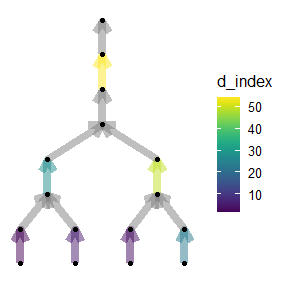# 5 References and key literature

Belletti, Barbara, et al. “More than one million barriers fragment Europe’s rivers.” Nature 588.7838 (2020): 436-441.

Cote, D., Kehler, D. G., Bourne, C., & Wiersma, Y. F. (2009). A new measure of longitudinal connectivity for stream networks. Landscape Ecology, 24(1), 101-113.

Kolaczyk, E. D., & Csárdi, G. (2014). Statistical analysis of network data with R (Vol. 65). New York: Springer.

Jumani, S., Deitch, M. J., Kaplan, D., Anderson, E. P., Krishnaswamy, J., Lecours, V., & Whiles, M. R. (2020). River fragmentation and flow alteration metrics: a review of methods and directions for future research. Environmental Research Letters.